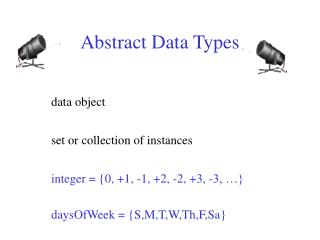DownloadDownload PresentationAbstract Data Types

# Abstract Data Types

Download Presentation## Abstract Data Types

- - - - - - - - - - - - - - - - - - - - - - - - - - - E N D - - - - - - - - - - - - - - - - - - - - - - - - - - -
##### Presentation Transcript

1. Abstract Data Types data object set or collection of instances integer = {0, +1, -1, +2, -2, +3, -3, …} daysOfWeek = {S,M,T,W,Th,F,Sa}

2. Data Object instances may or may not be related myDataObject = {apple, chair, 2, 5.2, red, green, Jack}

3. Data Structure Data object + relationships that exist among instances and elements that comprise an instance Among instances of integer 369 < 370 280 + 4 = 284

4. Data Structure Among elements that comprise an instance 369 3 is more significant than 6 3 is immediately to the left of 6 9 is immediately to the right of 6

5. Data Structure The relationships are usually specified by specifying operations on one or more instances. add, subtract, predecessor, multiply

6. Linear (or Ordered) Lists instances are of the form (e0, e1, e2, …, en-1) where ei denotes a list element n >= 0 is finite list size isn

7. Linear Lists L = (e0, e1, e2, e3, …, en-1) relationships e0 is the zero’th (or front) element en-1 is the last element eiimmediately precedes ei+1

8. Linear List Examples/Instances Students in MyClass = (Jack, Jill, Abe, Henry, Mary, …, Judy) Exams in MyClass = (exam1, exam2, exam3) Days of Week = (S, M, T, W, Th, F, Sa) Months = (Jan, Feb, Mar, Apr, …, Nov, Dec)

9. Linear List Operations—Length() determine number of elements in list L = (a,b,c,d,e) length = 5

10. Linear List Operations—Retrieve(theIndex) retrieve element with given index L =(a,b,c,d,e) Retrieve(0) = a Retrieve(2) = c Retrieve(4) = e Retrieve(-1) = error Retrieve(9) = error

11. Linear List Operations—IndexOf(theElement) determine the index of an element L =(a,b,d,b,a) IndexOf(d) = 2 IndexOf(a) = 0 IndexOf(z) = -1

12. Linear List Operations—Delete(theIndex) delete and return element with given index L = (a,b,c,d,e,f,g) Delete(2)returns c and L becomes (a,b,d,e,f,g) index ofd,e,f,andgdecrease by 1

13. Linear List Operations—Delete(theIndex) delete and return element with given index L = (a,b,c,d,e,f,g) Delete(-1)=> error Delete(20) => error

14. Linear List Operations—Insert(theIndex, theElement) insert an element so that the new element has a specified index L = (a,b,c,d,e,f,g) Insert(0,h)=> L = (h,a,b,c,d,e,f,g) index ofa,b,c,d,e,f,andgincrease by 1

15. Linear List Operations—Insert(theIndex, theElement) L = (a,b,c,d,e,f,g) Insert(2,h)=> L = (a,b,h,c,d,e,f,g) index ofc,d,e,f,andgincrease by 1 Insert(10,h) => error Insert(-6,h) => error

16. Data Structure Specification • Language independent • Abstract Data Type

17. Linear List Abstract Data Type AbstractDataTypeLinearList { instances ordered finite collections of zero or more elements operations IsEmpty(): return true iff the list is empty, falseotherwise Length(): return the list size (i.e., number of elements in the list) Retrieve(index): return theindexth element of the list IndexO f(x): return the index of the first occurrence of xin the list, return -1 ifxis not in the list Delete(index): remove and return theindexth element, elements with higher index have their index reduced by 1 Insert(theIndex, x): insert x as the indexth element, elements with theIndex >= indexhave their index increased by1 }